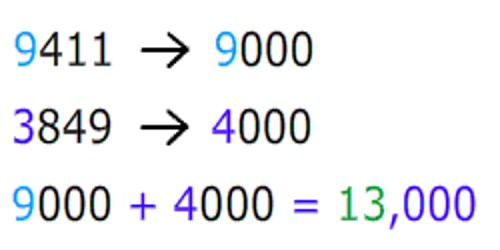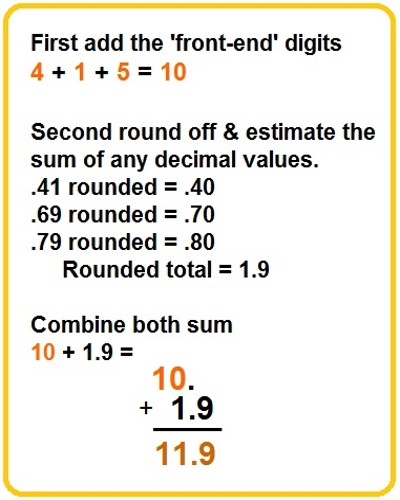Mathematic

# How to estimate a Sum by Front End Estimation?Front end estimation mostly produces a closer estimate of sums or differences than the answer produced by adding or subtracting rounded numbers. When finding the sum of several numbers, one way to estimate the sum is called “front-end’ estimation.

In front end estimation of sum you add only the first digit of the numbers. The other digits are replace by zeros.

How to estimate a sum by front end estimation:

• Add the digits of the two highest place values
• Insert zeros for the other place values

Example 1: 4496 + 3745 is estimated to be 8100 by front end estimation (i.e. 4400 + 3700).

Example 2: 4496 + 745 is estimated to be 5100 by front end estimation (i.e. 4400 + 700).

Front end estimation is just one way of estimating an answer. You will need to round the number so that there is one digit in the front and all others become zeros. The name comes from the way that you round. Instead of rounding each number to a given place value, we round whatever number is in the front.Real life example:

Charlene and Margi went to a nearby restaurant for a quick lunch. The waitress handed them the bill with the price of each lunch, but forgot to add up the total. Estimate the total bill if Charlene’s lunch was \$3.75 and Margi’s lunch was \$4.29.

Analysis: Estimation is a good tool for making a rough calculation. There are many estimation strategies that you could use to estimate the sum of these decimals. Let’s look at the front-end strategy and the rounding strategy. We will round to the nearest tenth.

Information Source;